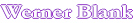Home                Vapor Pressure of Solvent Blends using Antoine Constants of Solvents

This program permits to calculate the vapor pressure of solvent blends at different temperatures using the Antoine equations and Raoult's law for solvent blends. The Antoine equation describes the relation between saturated vapor pressure and temperature of a pure component. The Antoine equation is derived from the Claussius-Clapeyron equation which describes the phase transition between two phases of matter.
For solvent blends the Raoult's law applies. The Raoult's law assumes that the solvent blend is uniformly mixed. Many solvent systems deviate from the linear behavior predicted by the law.
Antoine equation P = Pressure in Hg mm (torr), T =Temperature in şC,
P=10A-B/C+T) A, B, C = Antoine constants
Raoult's law Raoult's law states: the vapor pressure of an ideal solution is dependent on the vapor pressure of each chemical component and the mole fraction of the component present in the solution.
P=PAXA + PBXB + .... Vapor pressure P of a solvent blend is the sum of the partial pressures of the components
Pi=PiXi Pi is the vapor pressure of the pure component Xi is the mole fraction of the components
Enter Data

Results

Temperature, °C Moles Molar fraction Partial pressure Heat of vaporization
Weight     Hg mm  Hn, kJ/mol

Total
Vapor Pressure, Hg mm calc
Heat of Vaporization DHn, kJ/mol
Composition of Vapor Phase   Mol fraction

Weight

Weight fraction

Total
B.E.Poling, J.M.Prausnitz, YJ.P. O’Connell, The Properties of Gases and Liquids 5th Ed Appl A. Mc McGraw-Hill, New York NY, 2001
Antoine Parameters from Gmehling, J., Onken, U., and Arlt, W., Vapor-liquid Equilibrium Data Collection, Chemistry Data Series, Vol. I, Parts 1-8, DECHEMA (1977-1990).
 Last edited on: October 15, 2010 Copyright®, Design, Layout and Technical Content by:Automatic recalculation